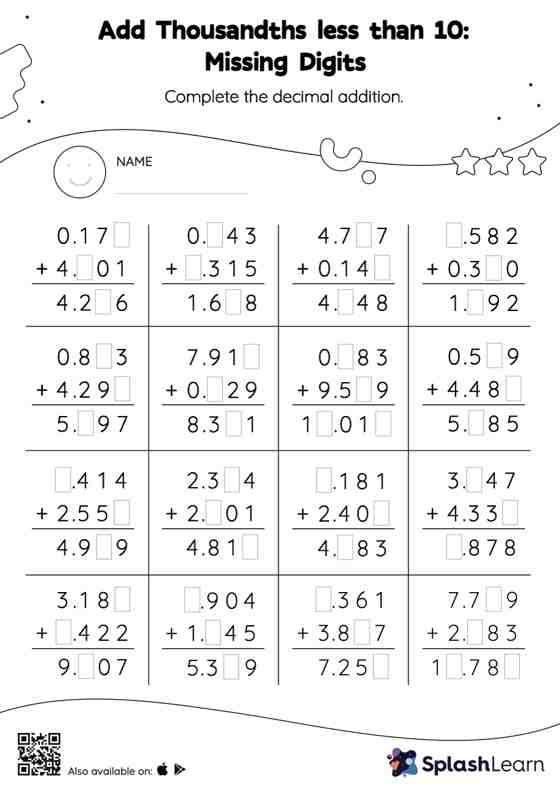# Add Thousandths less than 10: Missing Digits Worksheet

Home > Add Thousandths less than 10: Missing DigitsWhen adding decimals on this worksheet, students align the decimal points and use zero as a placeholder. Then to find the missing number in the add thousandths less than 10 worksheet, students then apply the relationship between addition and subtraction. In this worksheet, numbers are laid one on top of another (vertical format). This encourages students to use the inherent place value structure to solve the problems.